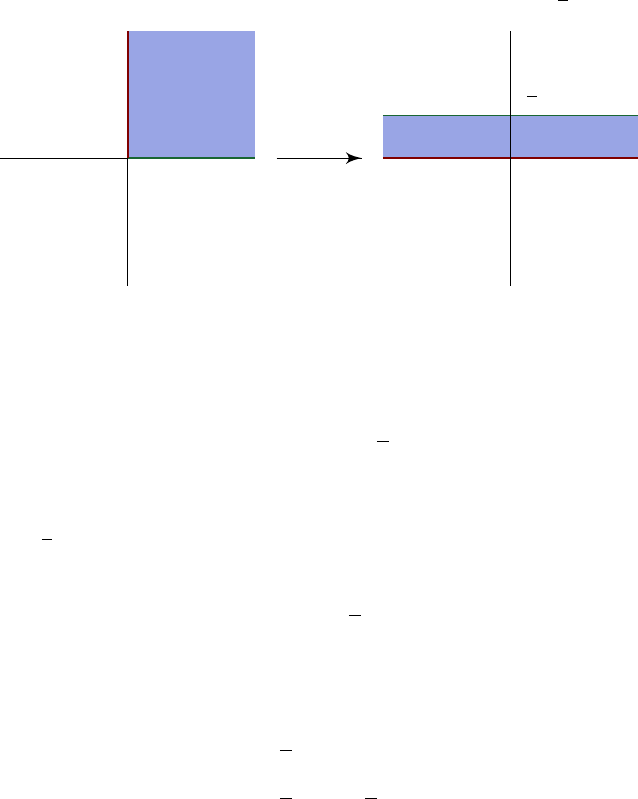1Analytic functions

IB Complex Methods1.7 Solving Laplace’s equation using conformal maps
As we have mentioned, conformal maps are useful for transferring problems from
a complicated domain to a simple domain. For example, we can use it to solve
Laplace’s equation, since solutions to Laplace’s equations are given by real and
imaginary parts of holomorphic functions.
More concretely, the following algorithm can be used to solve Laplace’s
Equation
2
φ
(
x, y
) = 0 on a tricky domain
U R
2
with given Dirichlet
boundary conditions on
U
. We now pretend
R
2
is actually
C
, and identify
subsets of R
2
with subsets of C in the obvious manner.
(i)
Find a conformal map
f
:
U V
, where
U
is now considered a subset of
C
, and
V
is a “nice” domain of our choice. Our aim is to find a harmonic
function Φ in V that satisfies the same boundary conditions as φ.
(ii)
Map the boundary conditions on
U
directly to the equivalent points on
V .
(iii) Now solve
2
Φ = 0 in V with the new boundary conditions.
(iv) The required harmonic function φ in U is then given by
φ(x, y) = Φ(Re(f(x + iy)), Im f(x + iy)).
To prove this works, we can take the
2
of this expression, write
f
=
u
+
iv
, use
the Cauchy-Riemann equation, and expand the mess.
Alternatively, we perform magic. Note that since Φ is harmonic, it is the
real part of some complex analytic function
F
(
z
) = Φ(
x, y
) +
i
Ψ(
x, y
), where
z
=
x
+
iy
. Now
F
(
f
(
z
)) is analytic, as it is a composition of analytic functions.
So its real part, which is Φ(Re f, Im f), is harmonic.
Let’s do an example. In this case, you might be able to solve this directly
just by looking at it, using what you’ve learnt from IB Methods. However, we
will do it with this complex methods magic.
Example.
We want to find a bounded solution of
2
φ
= 0 on the first quadrant
of R
2
subject to φ(x, 0) = 0 and φ(0, y) = 1 when, x, y > 0.
This is a bit silly, since our
U
is supposed to be a nasty region, but our
U
is
actually quite nice. Nevertheless, we still do this since this is a good example.
We choose f(z) = log z, which maps U to the strip 0 < Im z <
π
2
.
U
1
0
z 7→ log z
V
i
π
2
00
1
Recall that we said
log
maps an annulus to a rectangle. This is indeed the case
here
U
V
is an
infinitely long rectangle.
Now, we must now solve
2
Φ = 0 in V subject to
Φ(x, 0) = 0, Φ
x,
π
2
= 1
for all
x R
. Note that we have these boundary conditions since
f
(
z
) takes
positive real axis of
V
to the line
Im z
= 0, and the positive imaginary axis to
Im z =
π
2
.
By inspection, the solution is
Φ(x, y) =
2
π
y.
Hence,
Φ(x, y) = Φ(Re log z, Im log z)
=
2
π
Im log z
=
2
π
tan
1
y
x
.
Notice this is just the argument θ.